# 浅谈Kruskal算法

@小铭同学lym 2021-05-04 10:30 回复

## 2 做法

1. $\textit{Prim}$最小生成树算法。
2. $\textit{Kruskal}$最小生成树算法。

## 3 并查集

### 3-2 基本操作

1. 查找操作：

我们定义一个 $find$函数，作用是找x的祖先节点。就可以写出下面的程序：

int find(int x)
{
if(f[x]==x) \\如果它是祖先，就返回他
return x;
f[x]=find(f[x]);\\否则就找它的父亲的父亲是谁
return f[x];
}
2. 路径压缩

有人可能看不懂为什么要 f[x]=find(f[x]);

我们可以想一想，如果我们的要找某个节点的祖先。刚在 那棵树，是一个长长的链，那么我们的 $find$函数将会浪费大量的时间。导致超时。就像极了下面这张图：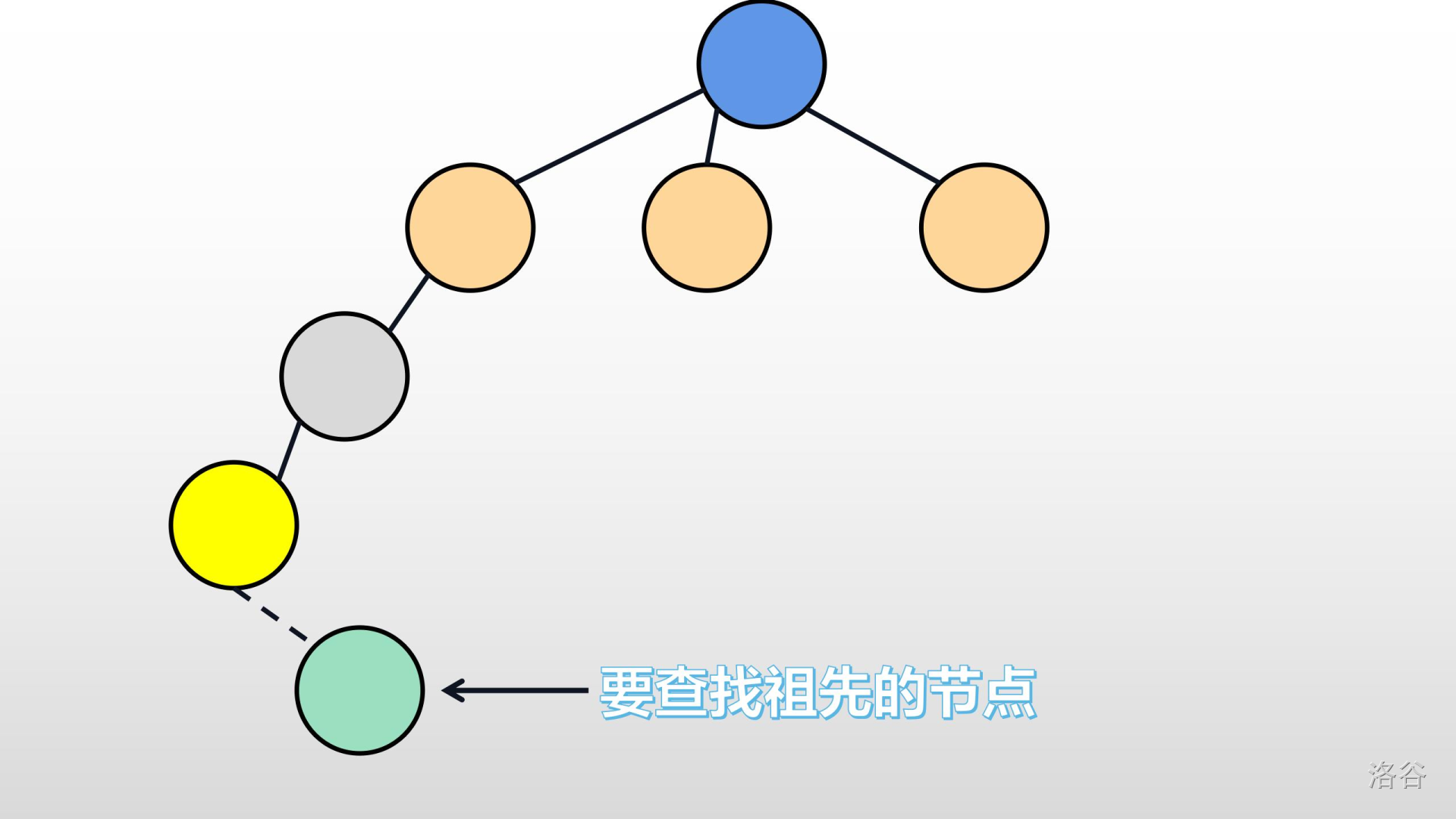其实有一个简单又行之有效的方法来解决：将每次需要找祖先的节点，找到祖先后，就讲那个节点的父亲设为它的祖先。因为我们完全不在意树的样子。这样，在下次再找的时候，就可以立即找到了。所以，我们可以这样做。就例如下图：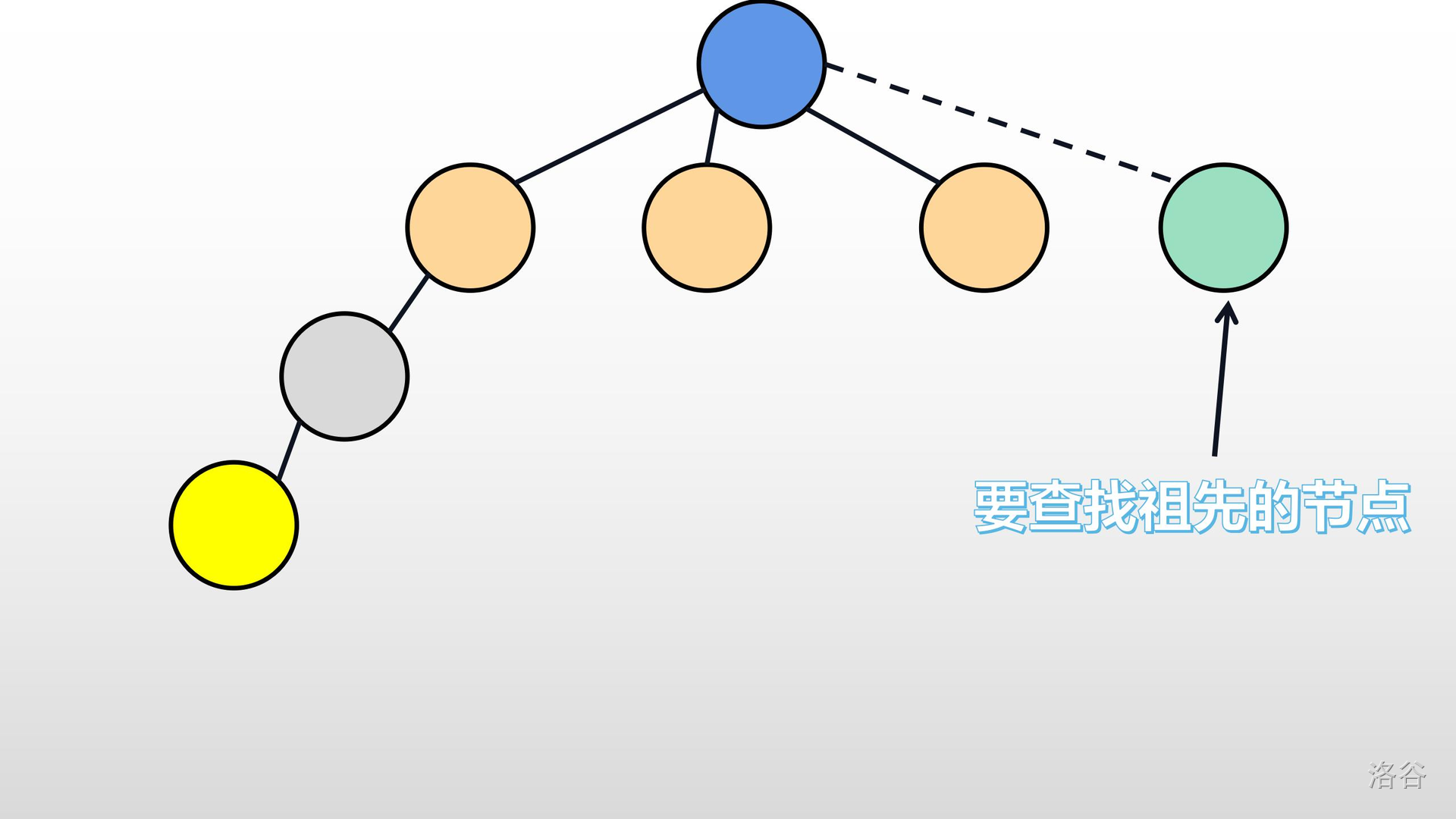至于图上的虚线，是为了更好的表达出怎么进行路径压缩。

3. 合并操作

现在我有一个问题想问你：如果我想要让之前两棵互不相干的数合并怎么办？或则说，我现在遇到节点 $y$，想让它属于 $x$，那一棵树，该怎么办？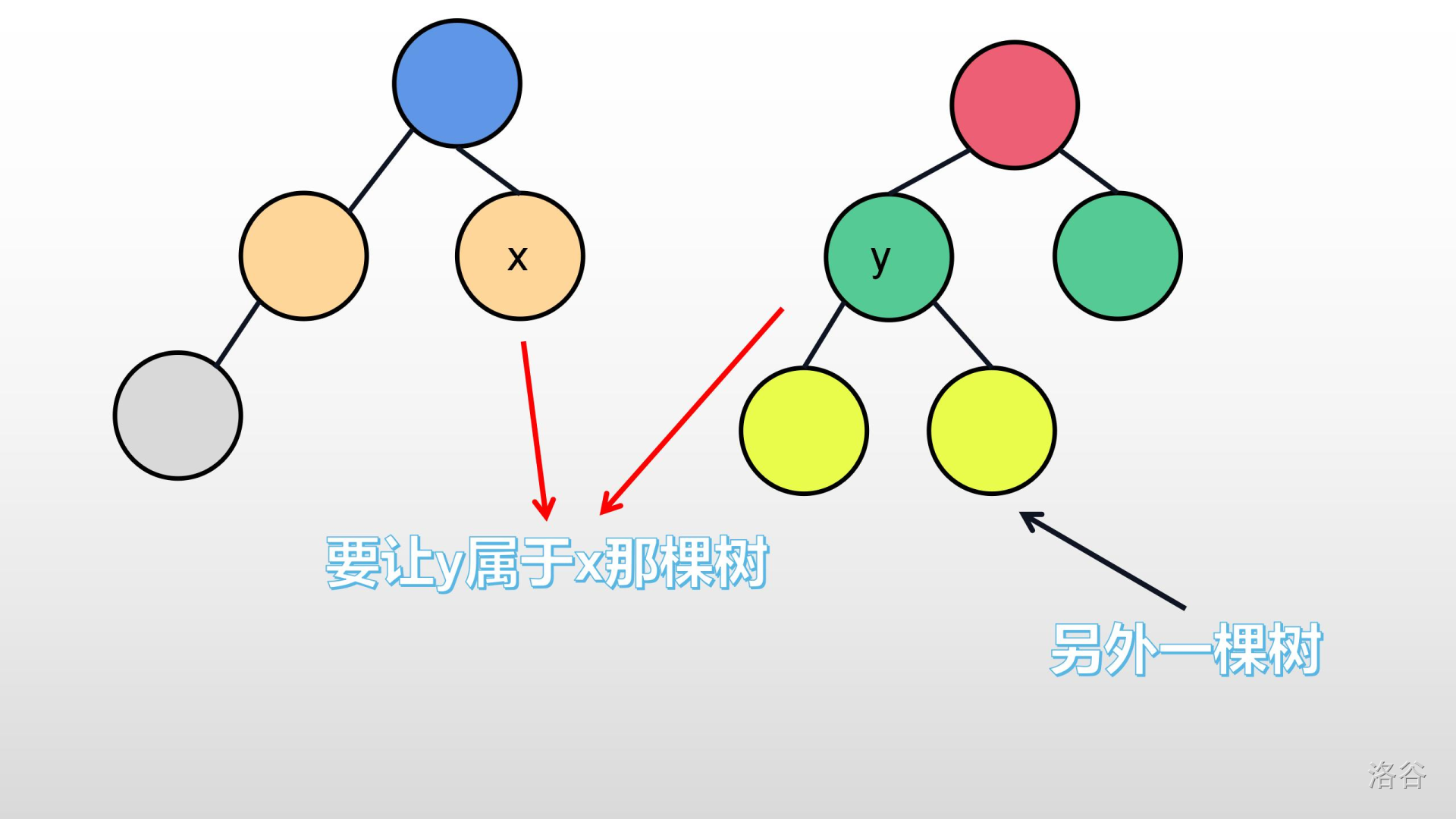有的人可能会直接将它们俩连起来，例如下图：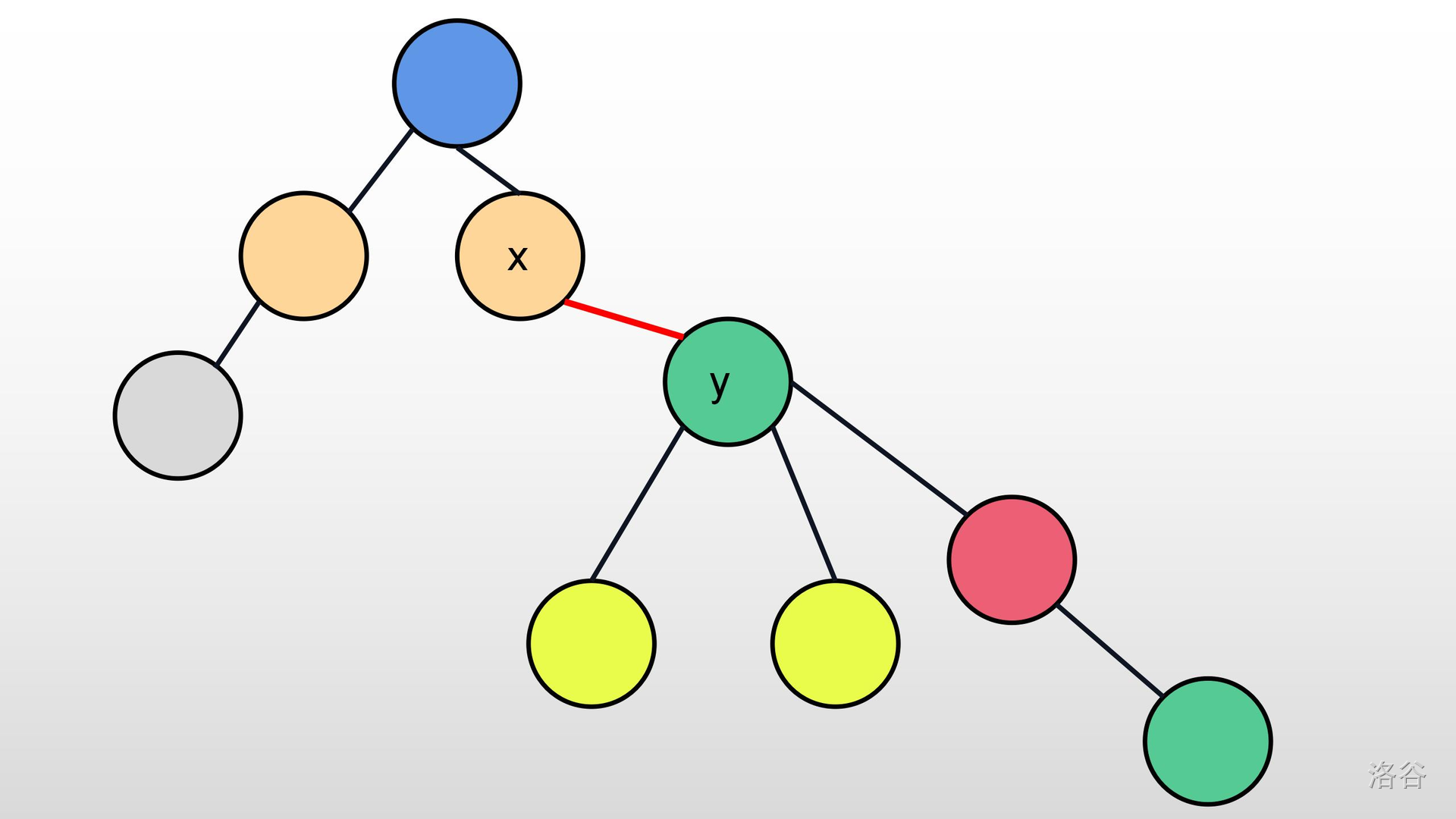但你是怎么把它们连起来的？直接让 $y$的父亲等于 $x$？也就是 $f[y]=x$？

但这样会发生什么？图中红色的点将会成为一个孤立的节点！我们可以仔细对比下面两个表格：

原来 父亲
y f[y]=红色节点
红色节点 f[红色节点]=它自己
现在 父亲
y f[y]=x
红色节点 f[红色节点]=它自己

也就是下图：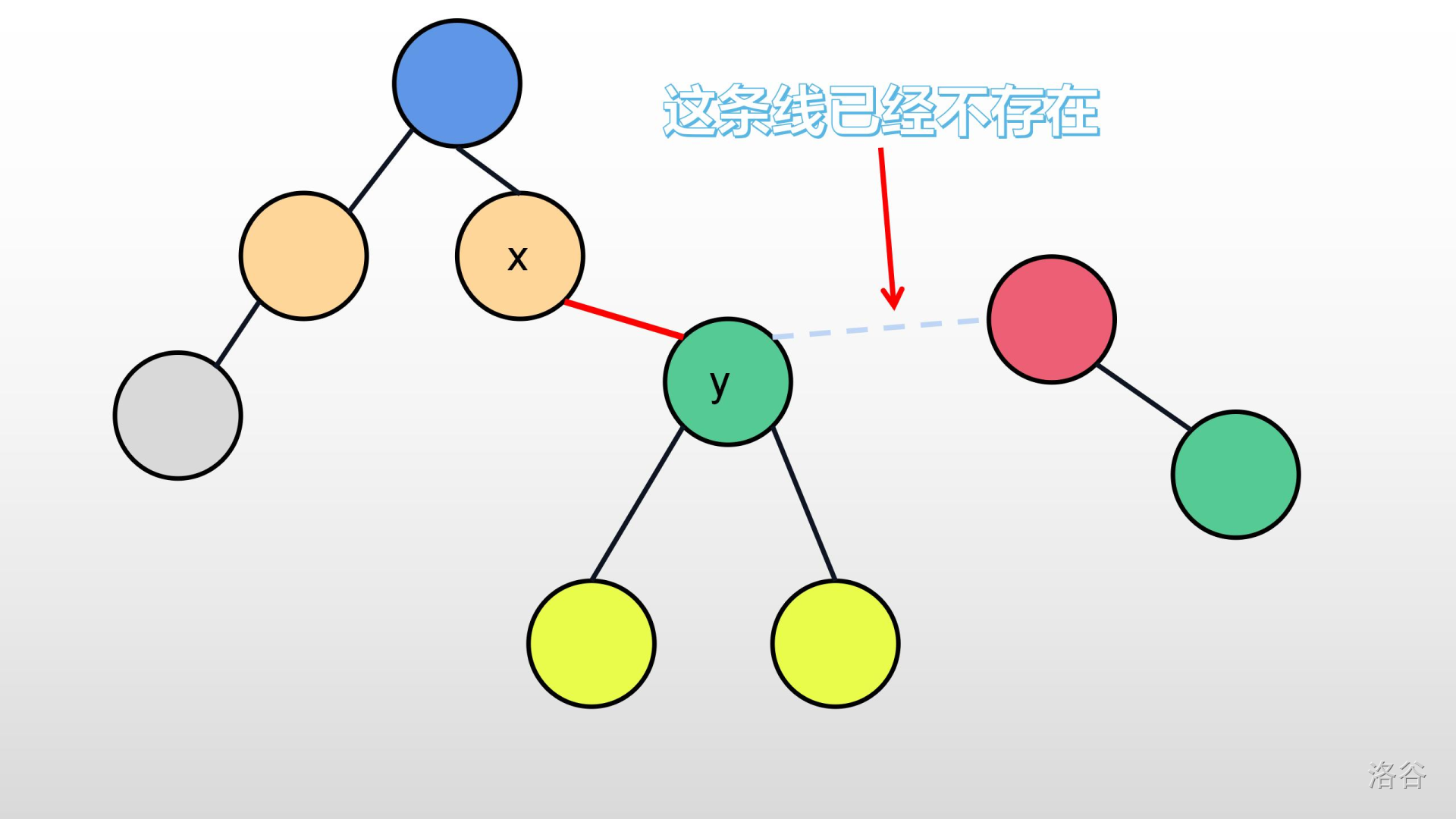那么我们需要考虑其他方法。

我们能不能让 $y$的祖先的父亲是 $x$的祖先呢？显然，是可以的。好比下图：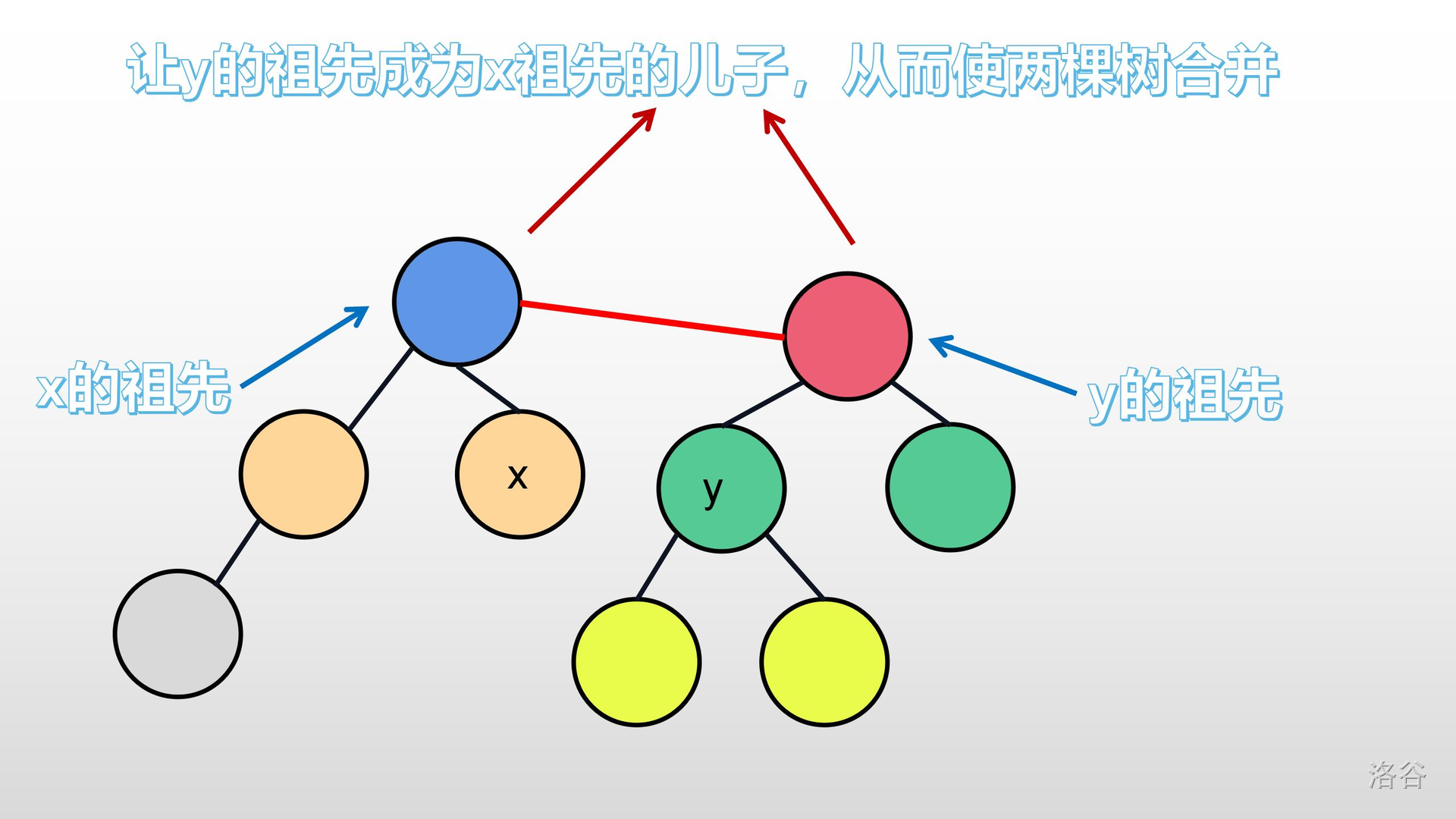所以，这个方法是可行的。

## 4 $\textit{Kruskal}$算法

### 4-1 步骤

1. 把所有的节点设为一个独立的集合；
2. 给边排序；
3. 选择新的节点是否能加入最小生成树中；
4. 把新的节点所在的树，与之前的树合并。

### 4-2 解释

1. 在这里，我们用结构体 $e[i].w$代表第 $i$条边的权值， $e[i].a$代表第 $i$条边的起点， $e[i].b$代表第 $i$条边的终点。
2. 为什么要把所有的节点设为一个独立的集合？

我们一开始还没有选择任何一条边，所以每一个节点都是一颗数（一个集合）；因此，把所有节点设为一个独立的集合。

3. 为什么给边排序？

因为我们是要选择权值和最小的生成树，当然与边所对应的权值有关了。而且，我们是要一颗生成树，我管你生出来是下面这样：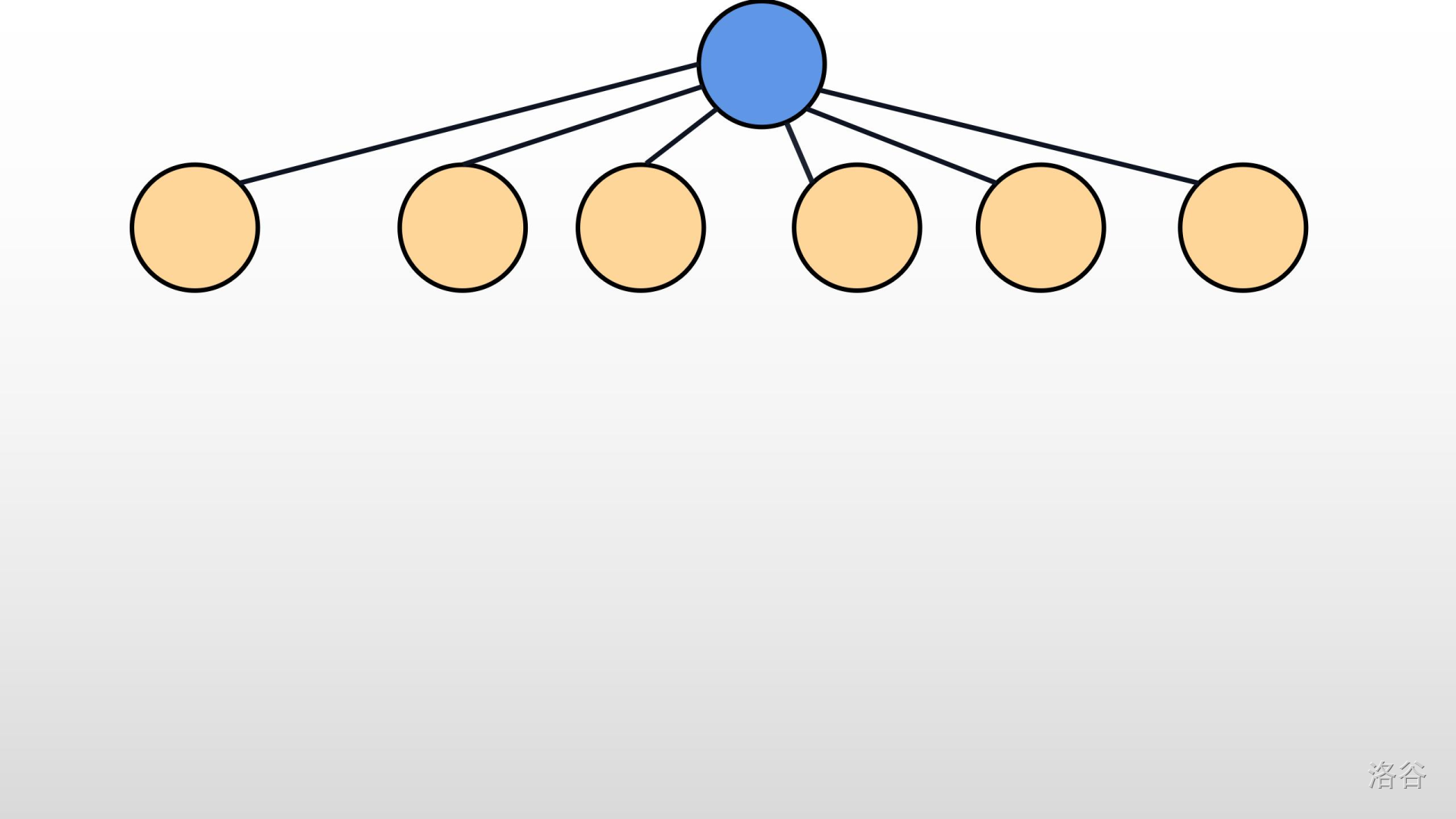还是下面这样：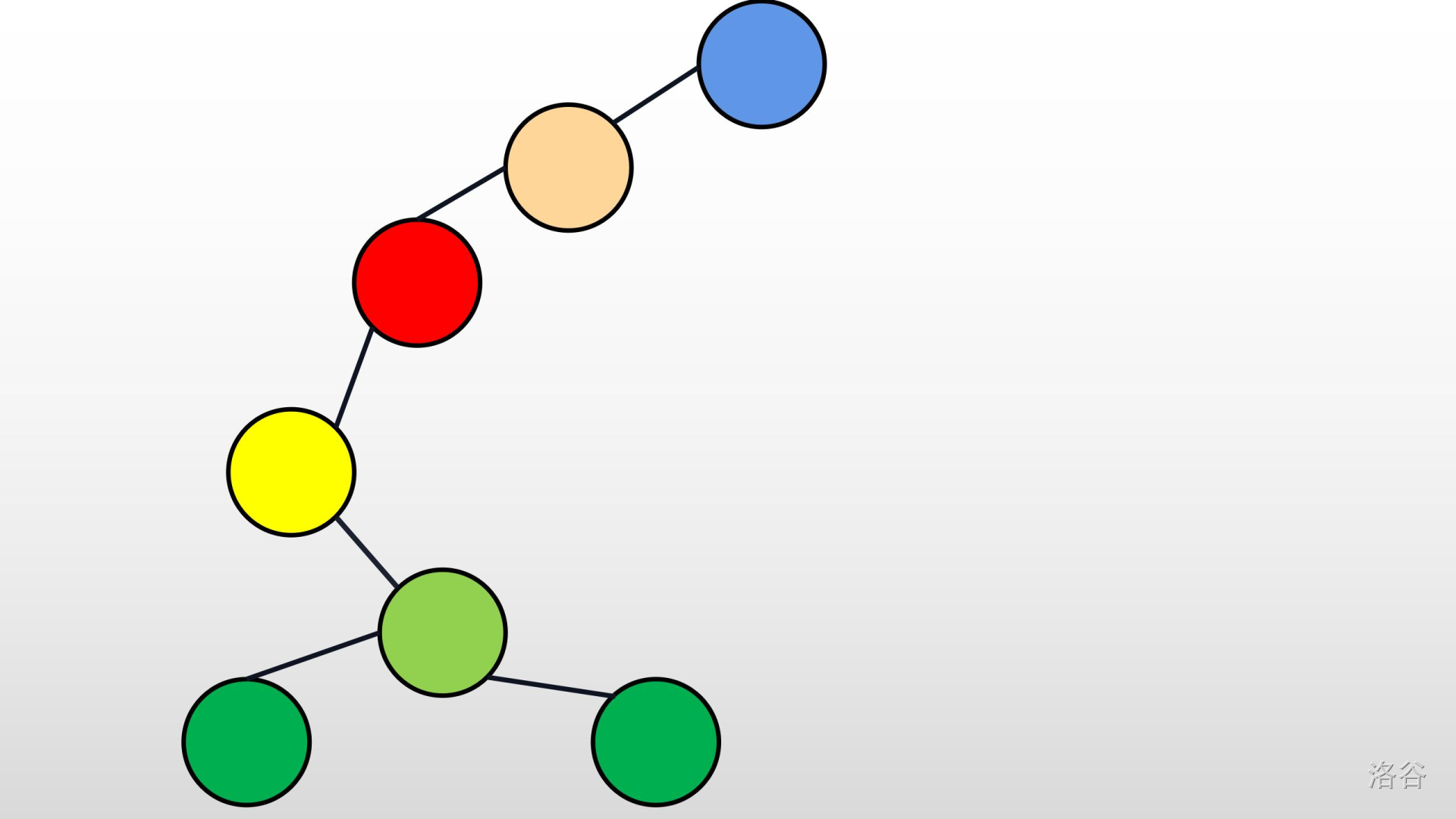只要生出来的是最小的就行了。

在这里，推荐用 $\textit{sort}$或 $\textit{qsort}$函数。而 $\textit{cmp}$函数是这样写的：

bool cmp(edge x,edge y)//这里的edge是结构体的名字。
{
return x.w<y.w;
}
4. 怎么判断是否能加入最小生成树？

好，我们都知道：树，里面是没有环的；如果有，那就是个图。那么，最小生成树里面，也是不允许有环的。如果两个节点已经在同一棵树里面，再把它们直接连起来，会形成一个环。就像下面这样：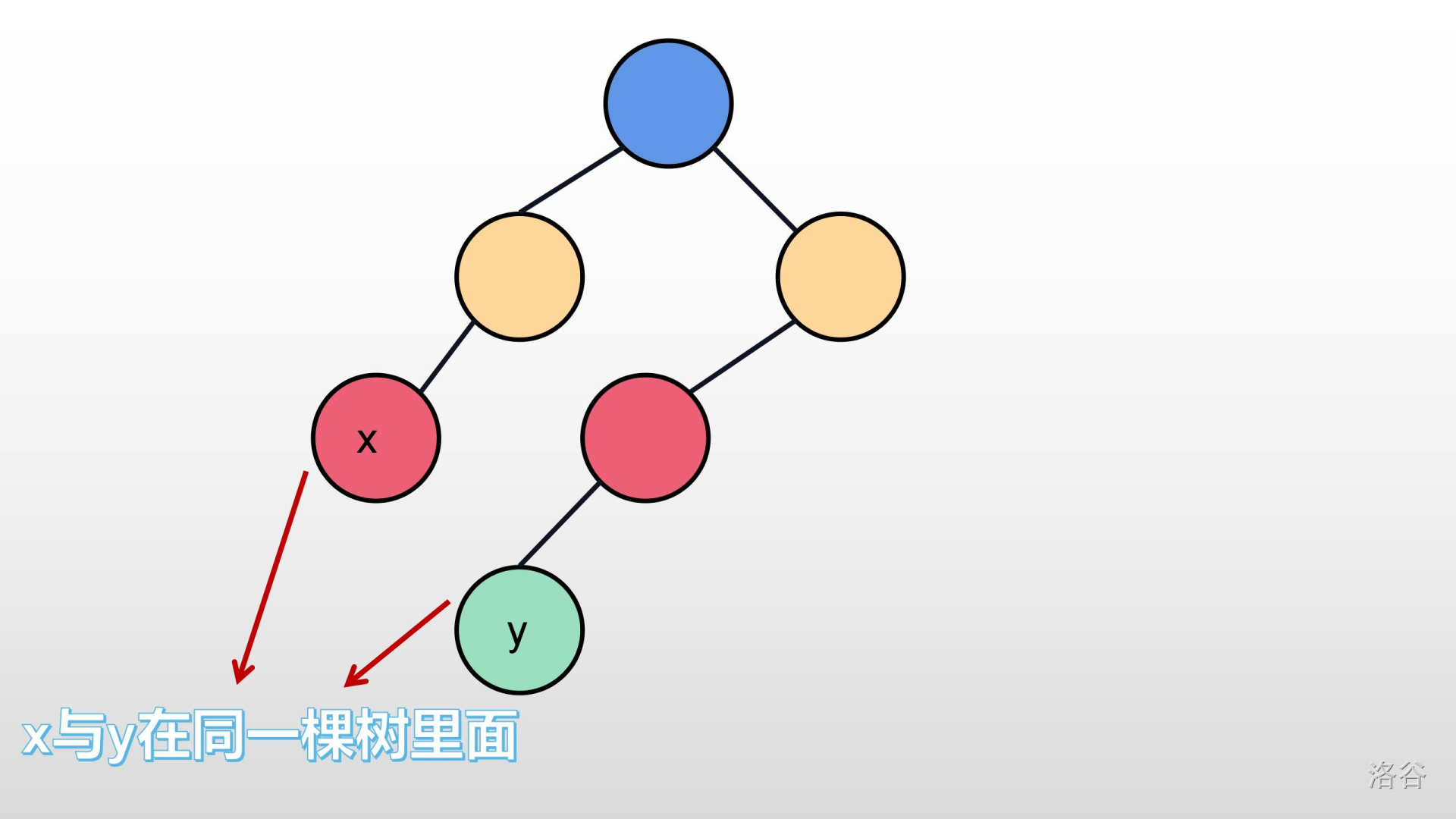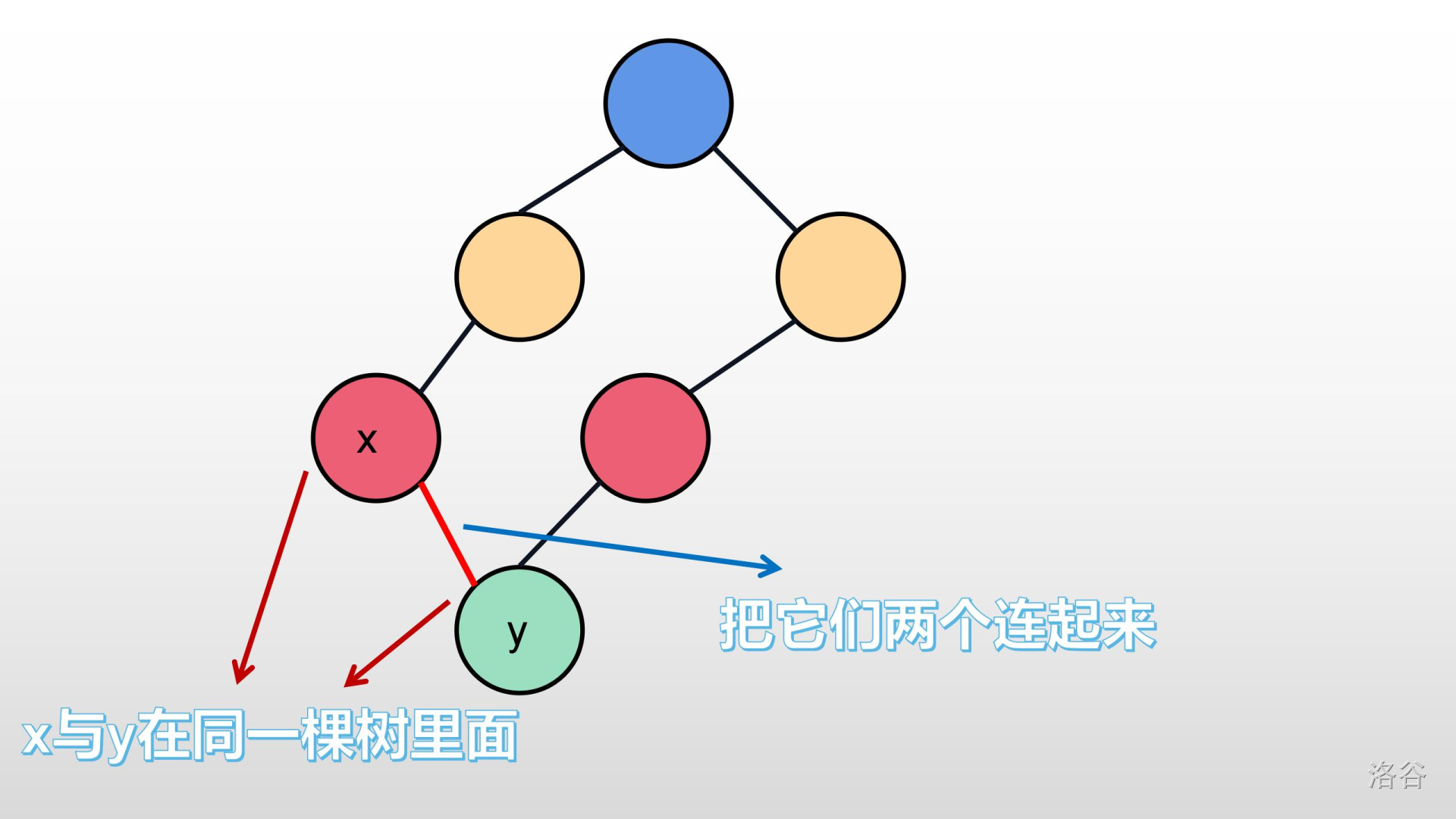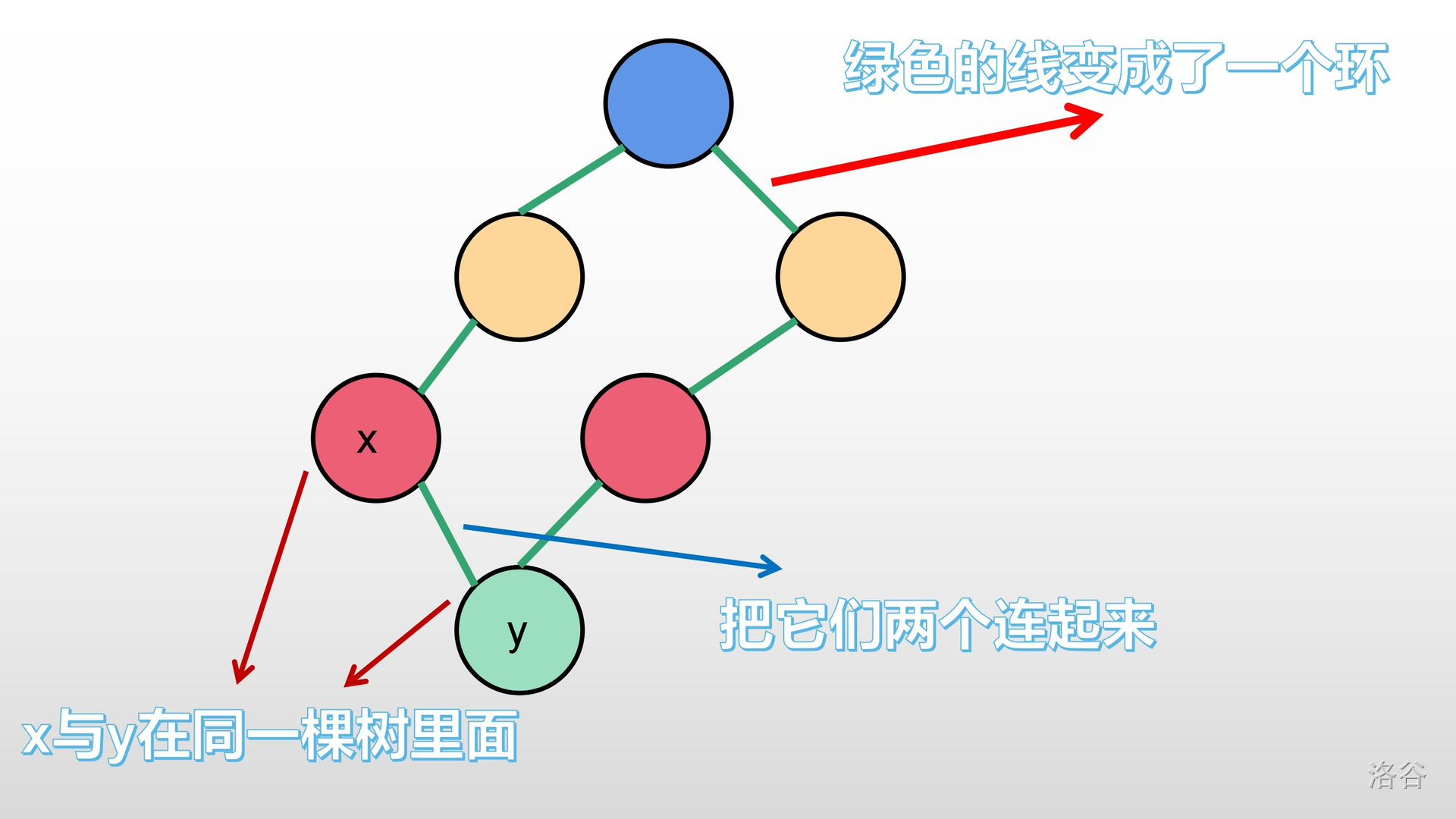所以我们不能让已经在同一棵树的两棵节点再连起来。这是，我们的并查集就有用了。

我们设 $x$存 $e[i].a$的祖先，也就是：

x=find(e[i].a);

我们设 $y$为 $e[i].b$的祖先，也就是：

y=find(e[i].a);

之后，我们再判断： $x$是否等于 $y$？如果是，就不把他们连起来；否则就把它们连起来。

这里，可能有人问：这又是为什么？

我们可以想一想，如果它们祖先相同，它们是不是在同一棵树内？如果把它们在连起来，岂不是变成了一张图？就完全和下面几张图一样：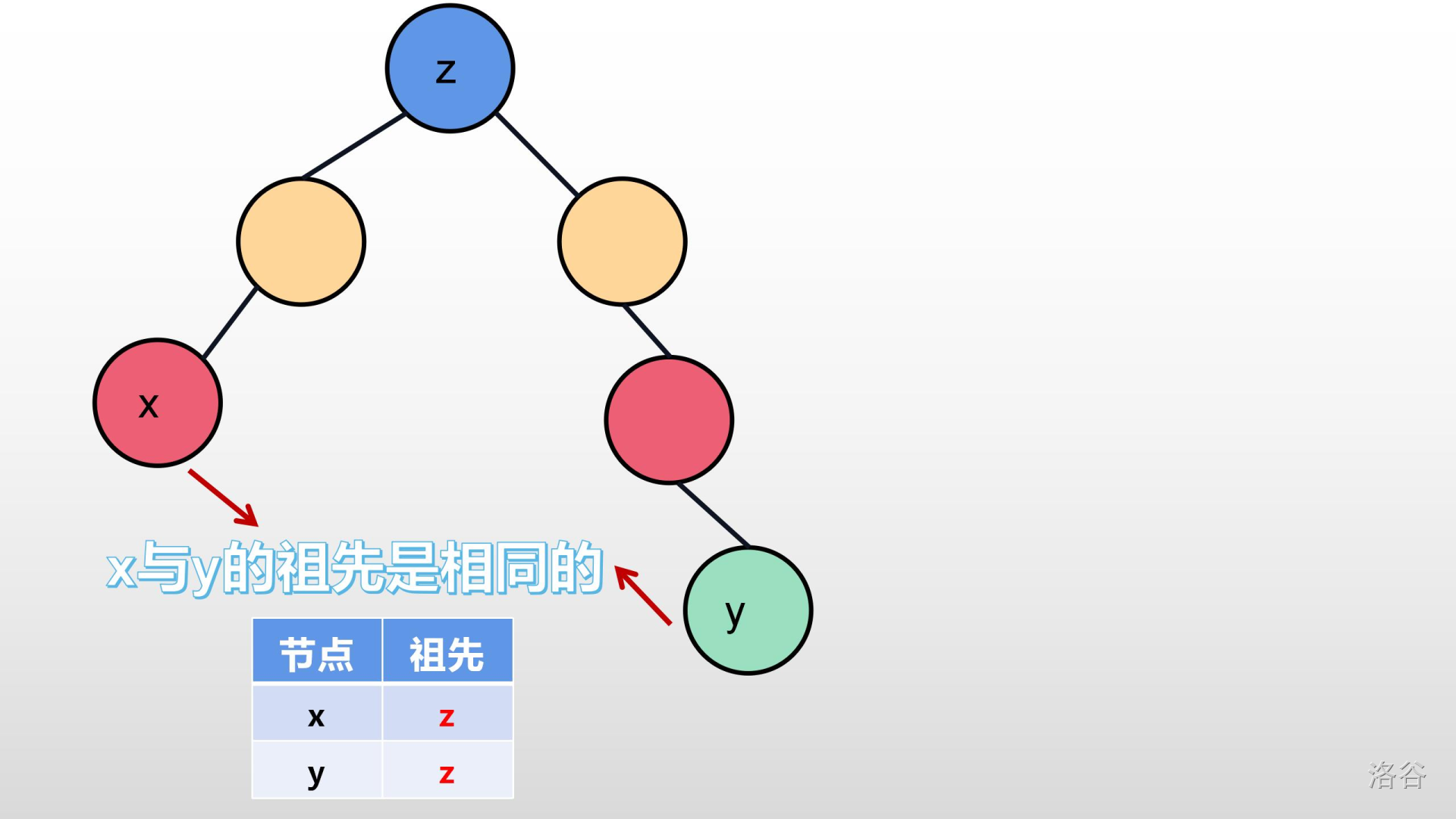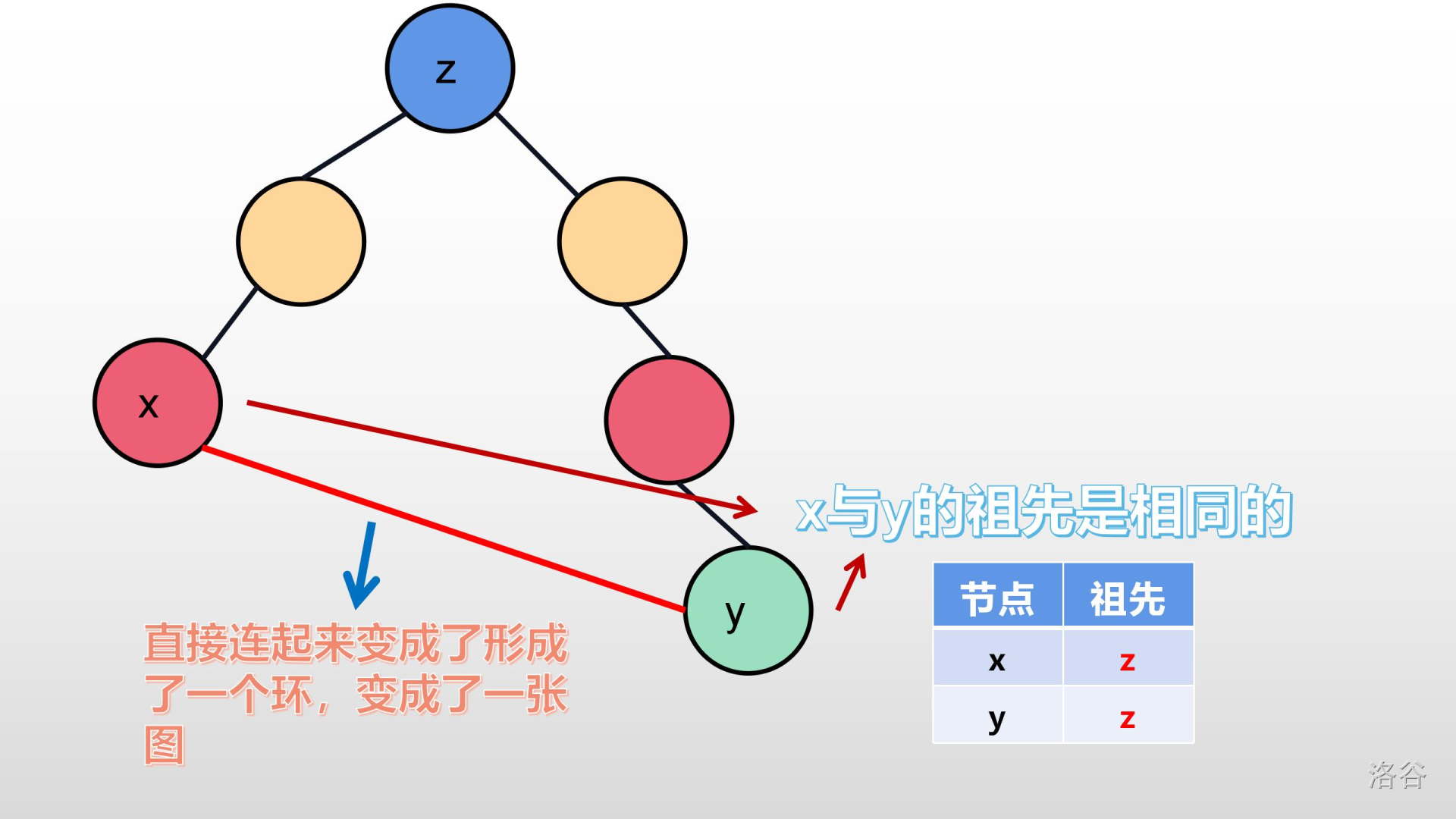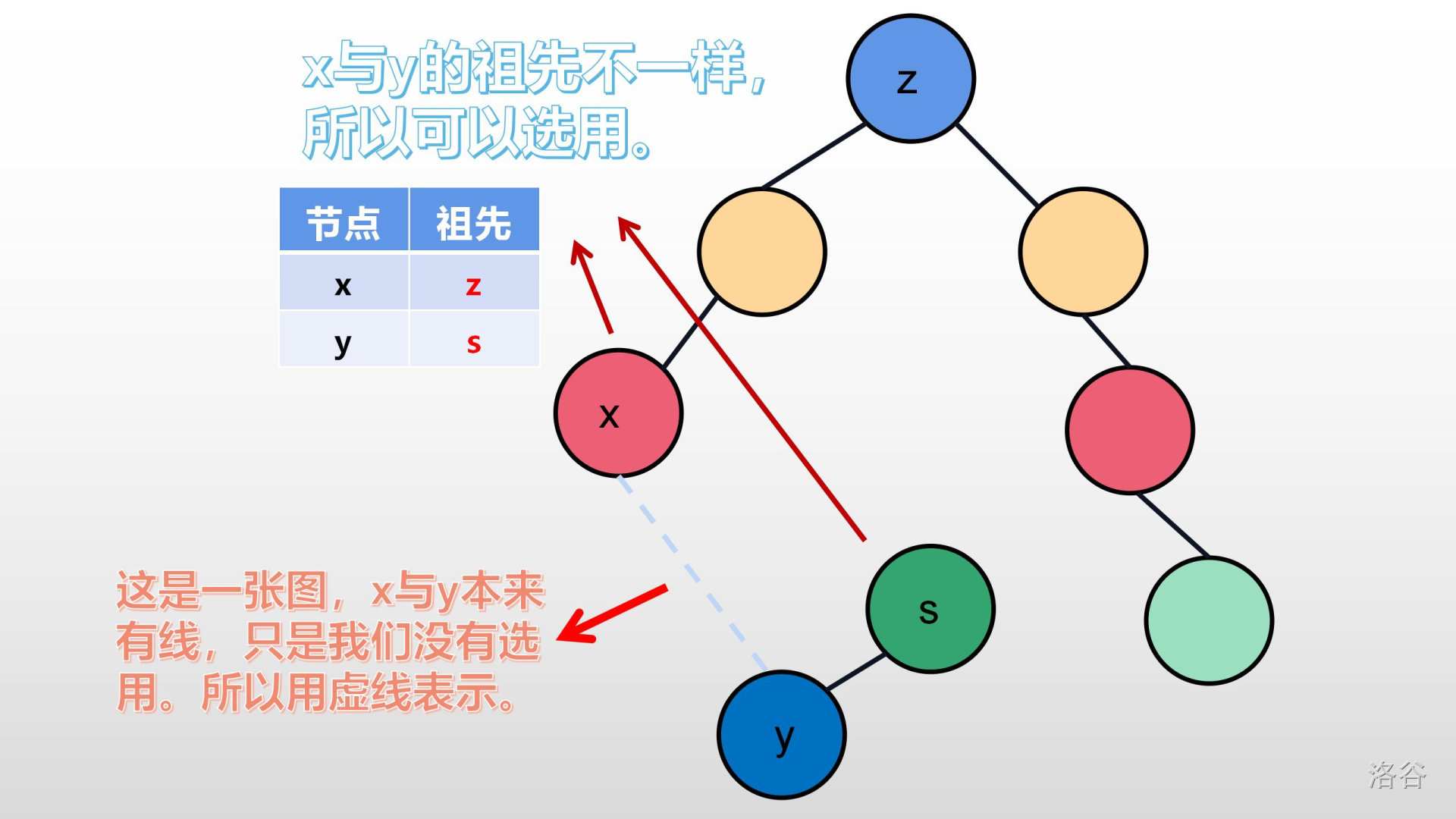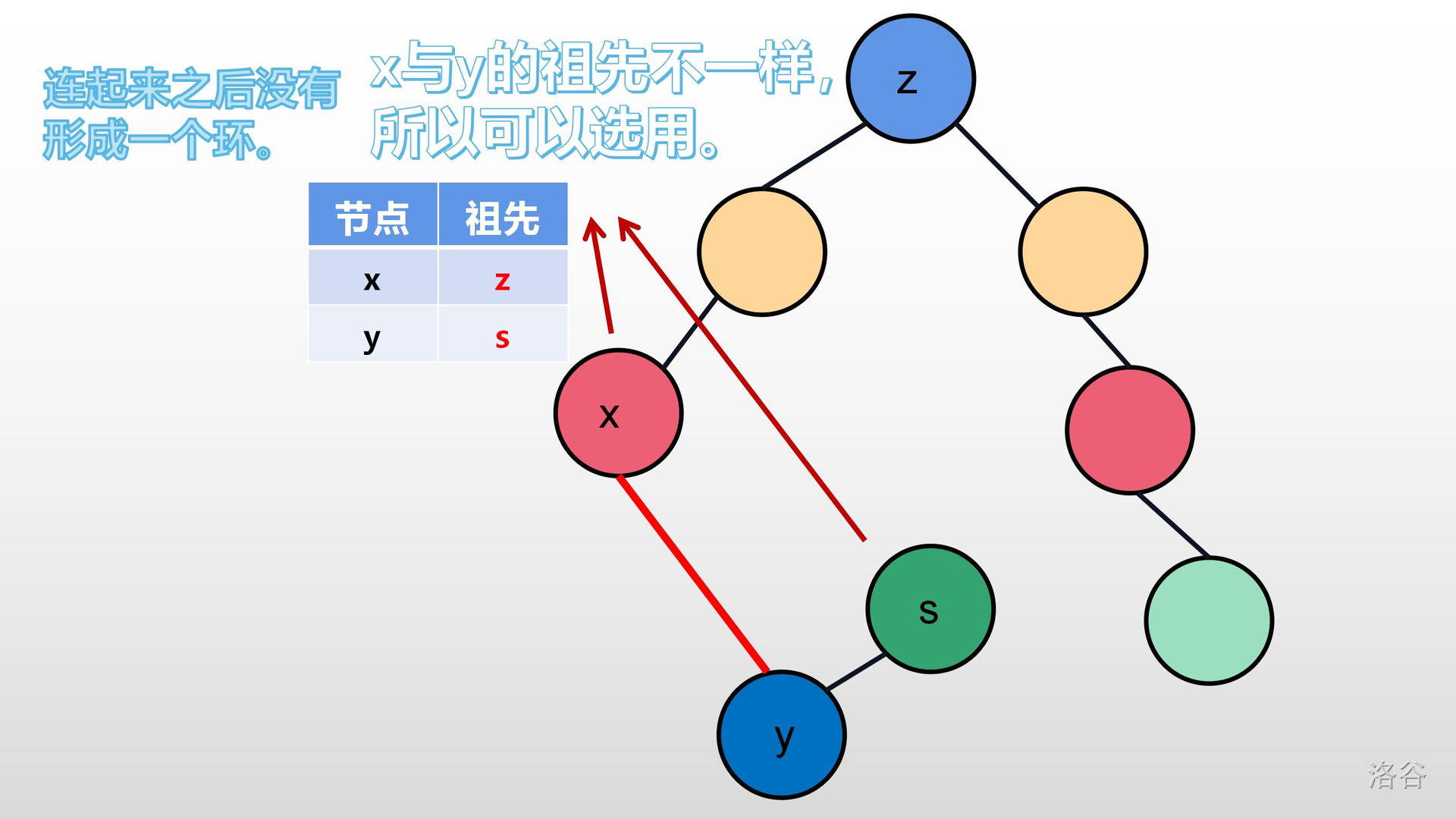在这里，有的人会问：加入了就是最优的？

我们可以思考一下：最小生成树是要连接所有的节点。图中已经有一个可以花最小权值（费用）的边去连接一个新的节点（或树），我们为什么不连？还是之前那句话：“我们是要一颗生成树，我们不需要去管生出来是怎样这样，只要管只要生出来的是最小的就行了。”

5. 为什么要让：把新的节点所在的树，与之前的树合并？怎么合并？

因为我们知道，它们如果连在了一起，它们就是同一棵树了（同一个集合）。所以我们要把它们给弄到一个集合，否则下次在找到这个树的时候，会重复。此时我们可以应用并查集合并功能。

让 $x$的祖先的父亲等于 $y$的祖先（或让 $y$的祖先的父亲等于 $x$的祖先），也就是：

f[find(x)]=find(y);

或者：

f[find(y)]=find(x);

## 5 具体程序和时间复杂度

### 5-1 具体程序

struct edge//结构体
{
int a,b,w;//a为起点，b为终点，w为权值
}e[maxm];//maxm为题目所说的最多的边数
bool cmp(edge x,edge y)//cmp函数
{
return x.w<y.w;
}
int find(int x)//并查集的查找函数（find函数）
{
if(f[x]==x)
return x;
return f[x]=find(f[x]);
}
int Kruskal()
{
for(int i=1;i<=n;i++)  f[i]=i;//一开始，所有的节点都是一个独立的集合（初始化）
sort(e+1,e+m+1,cmp);//排序
for(int i=1;i<=m;i++)//m为题目所给定的边数
{
int x=find(e[i].a);
int y=find(e[i].b);
if(x!=y)//如果可以合并新节点
{
ans+=e[i].w;//费用要加上
f[x]=y;//合并
}
}
}

## 6 适用范围

• $\textit{Kruskal }$适合用在稀疏图里面；
• $\textit{Prim }$适合用在稠密图里面。